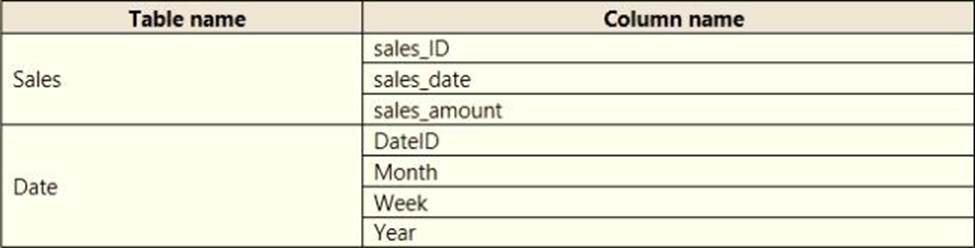Warning: Undefined variable \$in_footer in /var/www/html/exam4training.com/wp-content/themes/ultra-child/functions.php on line 14
Which DAX expression should you use? - Exam4Training

# Which DAX expression should you use?

You have a sales system that contains the tables shown in the following table.The Date table is marked as a date table.

DatelD is the date data type. You need to create an annual sales growth percentage measure.

Which DAX expression should you use?
A . SUM(sales[sales_amount]) – CALCULATE(SUM(sales[sales_amount]), SAMEPERIODLASTYEAR(‘Date'[DateID])
B . (SUM(‘Sales’[sales_amount]) – CALCULATE(SUM(‘Sales’[sales_amount]), SAMEPERIODLASTYEAR(‘Date’[DateID])))
/ CALCULATE(SUM(‘Sales’[sales_amount]), SAMEPERIODLASTYEAR(‘Date’[DateID]))

C . CALCULATE(SUM(sales[sales_amount]), DATESYTD(‘Date’[DateID]))
D . CALCULATE(SUM(sales[sales_amount]), SAMEPERIODLASTYEAR(‘Date’[DateID]))